# Pauli Matrices: What They Are and How to Prove the Commutation Relations Using FORTRAN90

Knoji reviews products and up-and-coming brands we think you'll love. In certain cases, we may receive a commission from brands mentioned in our guides. Learn more.
Pauli Spin matrices are 2X2 complex matrices which are very frequently used in quantum mechanics. They have some interesting characteristics. One of them is commutation relations. In this article I will prove these commutation relations using fortran90 w

Pauli Spin matrices are 2X2 complex matrices which appear very frequently in quantum mechanics. They are defined as: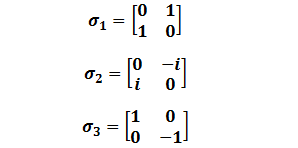Properties:

These matrices have some interesting properties, like

1) Squares of them give 2X2 identity matrices.

2) Determinant of Pauli matrices is -1.

3) Anti-commutation of Pauli matrices gives identity matrix when they are taken in cyclic order.

4) Commutation of two Pauli matrices gives another Pauli matrix multiplied by 2i (i is the imaginary unit) when they are taken in cyclic order.

Algebraic expressions make these properties clear.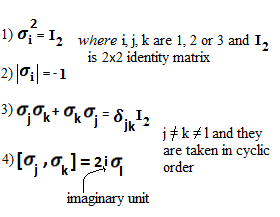In this article I will show how to prove the commutation relation in fortran90 which is a very popular and easy programming language.

Preparation for doing Programming:

Before starting the attempt of doing the program we need to know

i)What does ‘commutation’ means?

Commutation of A and B means [A, B] = AB-BA where A and B are operator or matrices. It is very much obvious that Commutation of two numbers (real or complex) is zero. Commutation of two matrices can also be zero but that is not true for all matrices. Again commutation of two operators can also vanish. I am not going to discuss that in this article.

ii) How to work with complex numbers in fortran90?

It is very easy to handle complex numbers in fortran90. Complex number is defined there as a data type. We have to enter a complex number in program like (1.0, 2.0) = 1+2i, (3.3, 0.0) = 3.3, (0.0, 2.7) = 2.7i etc.

An example of using array of complex numbers is illustrated below and the output is also given to make clear that how to handle complex numbers and matrix with complex elements: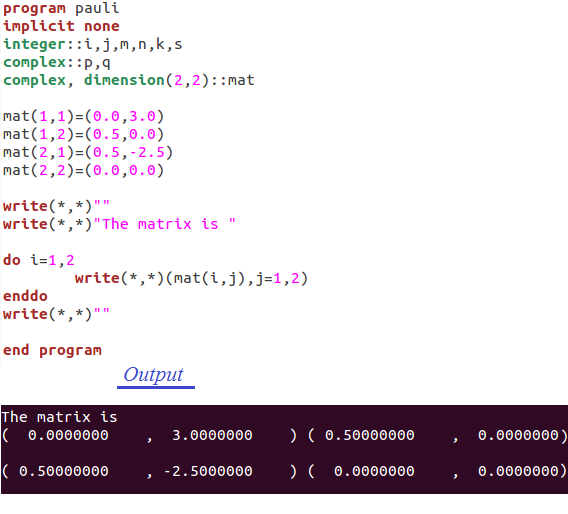iii) How to multiply two matrices in fortran90?

As we all know, for the multiplication of two matrices we have to have two matrices such that the number of columns of the first matrix is same as the number of rows of the second matrix. Symbolically Cij=Aik*Bkj where k varies from 1 to the number of columns of first matrix (or number of rows of second matrix). Let us do a program to learn multiplication of two matrices.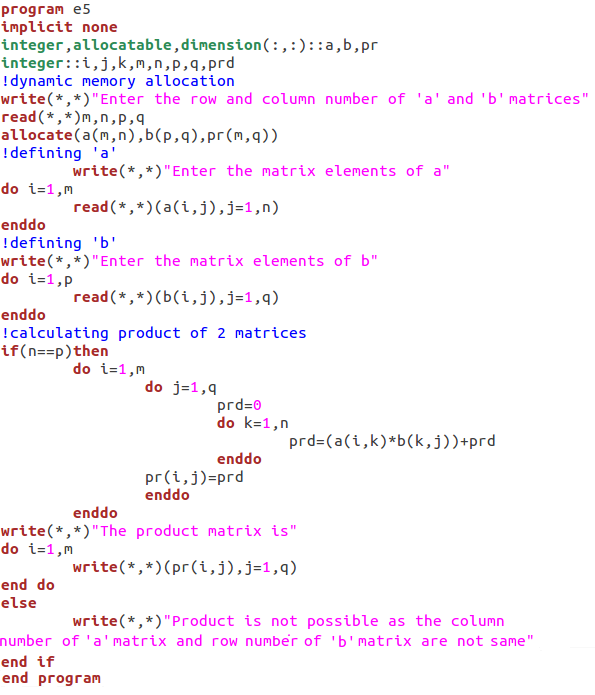Now we can proceed to do the programming to prove the commutation relation of Pauli matrices. Here I will only show the proof of the property (4) with j=1,k=2,l=3 only as the other relations are very much similar to show.

Programming:

Here is our fortran90 programming for proving that property and output is also given.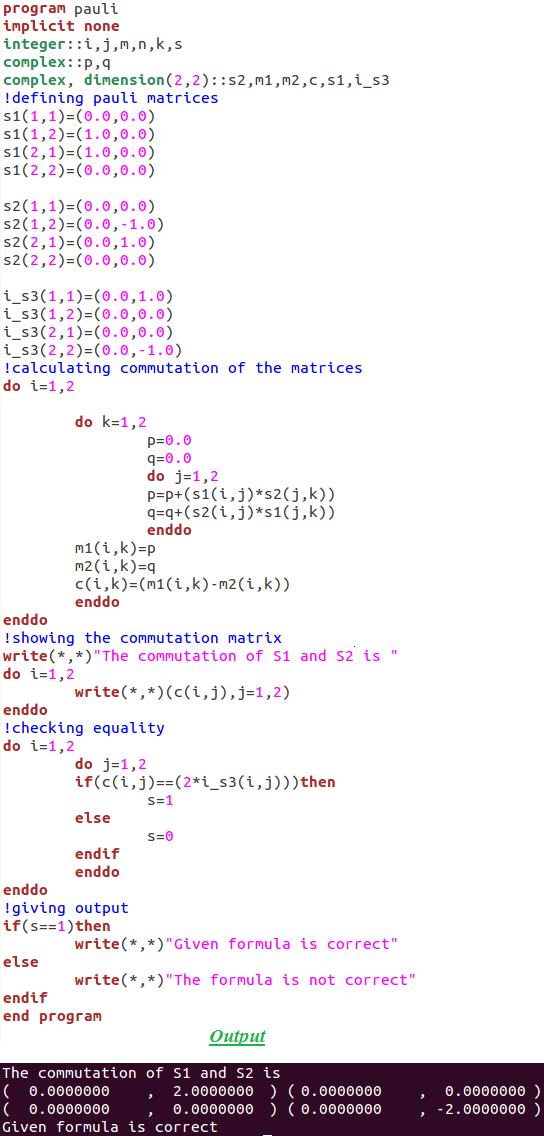Thus we see how to handle Pauli spin matrices in fortran90 hence learn performing complex arithmetic.